# Java iteration statements Iteration statements are statements which

• Slides: 33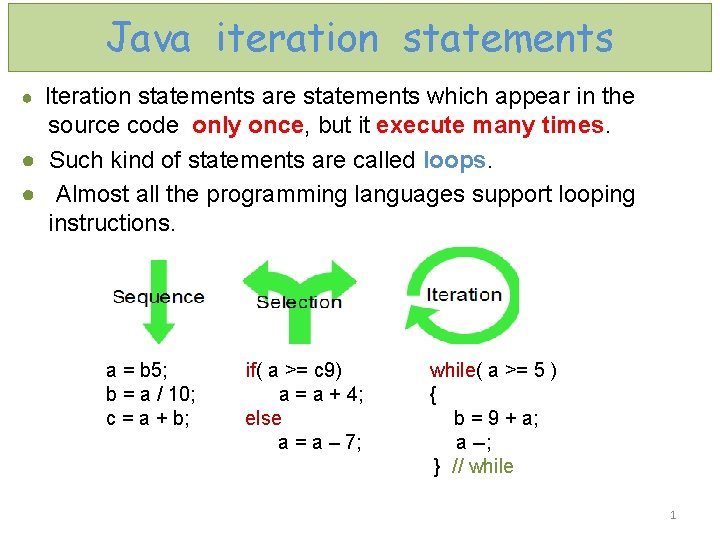Java iteration statements ● Iteration statements are statements which appear in the source code only once, but it execute many times. ● Such kind of statements are called loops. ● Almost all the programming languages support looping instructions. a = b 5; if( a >= c 9) while( a >= 5 ) b = a / 10; a = a + 4; { c = a + b; else b = 9 + a; a = a – 7; a --; } // while 1Iteration statements - Loops Java has three kinds of iteration statements ( לולאות / חזרה ) הוראות : WHILE loop FOR loop DO. . . WHILE loop Iteration (repetition) statements causes Java to execute one or more statements as long as a condition exist. Each repetition statement request : 1. a control variable / loop counter ( משתנה הלולאה / ) מונה הלולאה 2. the initial value of control variable 3. the increment (decrement) by which the control variable is modified each time through the loop 4. the loop continuation condition that determines if looping should continue. 2Basic elements of iterations /* example with the while statement prints numbers from 1 through 10 */ public class While_Test 1 { public static void main(String[ ] args) { Declare and initialize control variable int counter = 1; while(counter <= 10) Loop continuation condition { System. out. print(counter); counter++; } // while Increment control variable by 1 System. out. println(); } // main } // class 3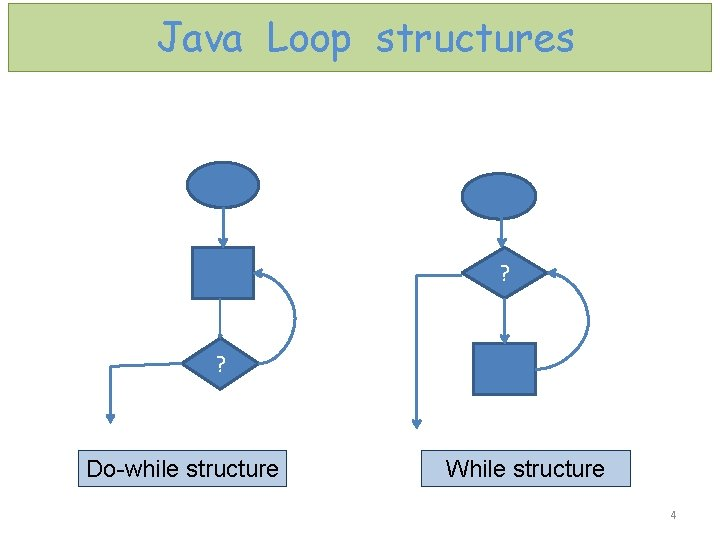Java Loop structures ? ? Do-while structure While structure 4While loop statement int count = 0; while (loop-continuation-condition) { while (count < 100) { // loop-body; System. out. println("Welcome to Java!"); Statement(s); count++; } //while } // while 5This program section reads a series of integers and computes their average. We designate zero to be a sentinel ( )זקיף value that indicates the end of the input. A sentinel must always be outside the normal range of values entered. int sum = 0, num, count = 0; // sum of series, input variable and loop counter double avg; // average of series System. out. println(“enter an integer ( 0 to quit) : “); num = reader. next. Int(); Sentinel value of 0 to terminate loop. while (num != 0) Sentinel value is not counted. { count++; sum+ = num; System. out. println(“enter an integer ( 0 to quit) : “); num = reader. next. Int( ); Casting } // while avg = (double)sum/count; System. out. println(“The average is : “ + avg); 6While loop example 1 • Input : – Two integers – num 1 and num 2 • Output : – How many times num 1 contains num 2 This is the result of the integer division num 1/num 2 • Note : – Do not use the division operator ( / ) ! 7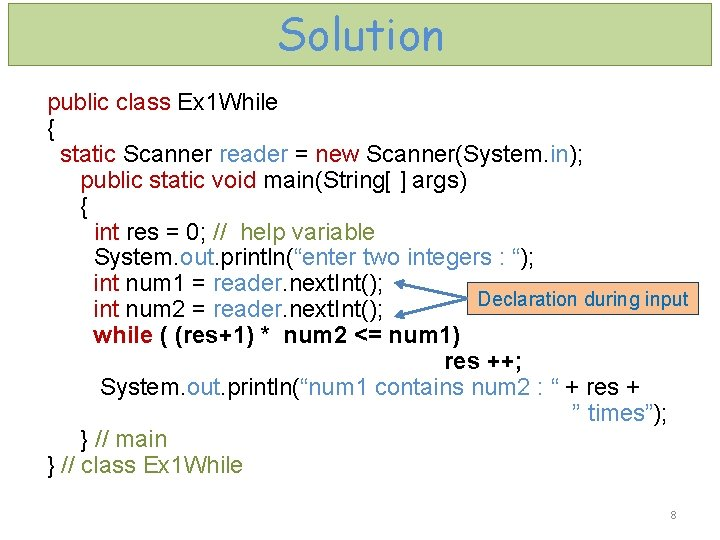Solution public class Ex 1 While { static Scanner reader = new Scanner(System. in); public static void main(String[ ] args) { int res = 0; // help variable System. out. println(“enter two integers : “); int num 1 = reader. next. Int(); Declaration during input int num 2 = reader. next. Int(); while ( (res+1) * num 2 <= num 1) res ++; System. out. println(“num 1 contains num 2 : “ + res + ” times”); } // main } // class Ex 1 While 8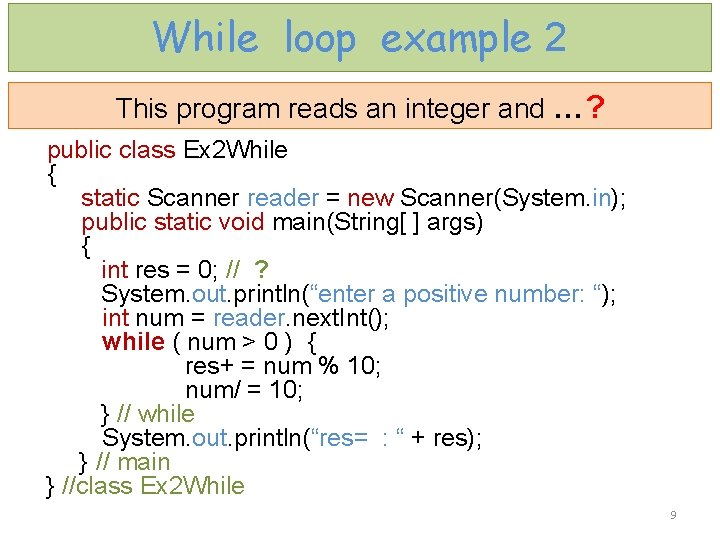While loop example 2 This program reads an integer and …? public class Ex 2 While { static Scanner reader = new Scanner(System. in); public static void main(String[ ] args) { int res = 0; // ? System. out. println(“enter a positive number: “); int num = reader. next. Int(); while ( num > 0 ) { res+ = num % 10; num/ = 10; } // while System. out. println(“res= : “ + res); } // main } //class Ex 2 While 9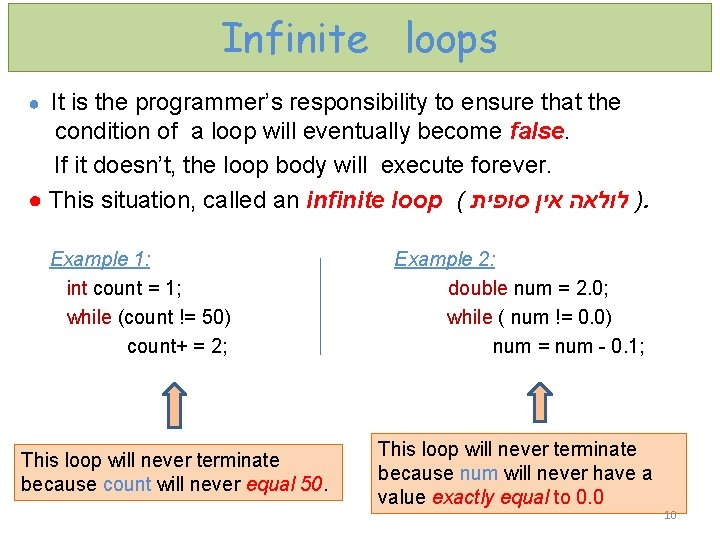Infinite loops ● It is the programmer’s responsibility to ensure that the condition of a loop will eventually become false. If it doesn’t, the loop body will execute forever. ● This situation, called an infinite loop ( ) לולאה אין סופית. Example 1: Example 2: int count = 1; double num = 2. 0; while (count != 50) while ( num != 0. 0) count+ = 2; num = num - 0. 1; This loop will never terminate because count will never equal 50. This loop will never terminate because num will never have a value exactly equal to 0. 0 10FOR loop statement • Equivalent to while… Any for loop can be converted to while loop and vice versa. • If we want to perform something for a predefined number of times, better use for. • If we just wait for something to happen (not after a certain number or iterations), better use while. • The for loop has three expressions that are contained within parentheses and separated with a semicolon. 11FOR loop - structure program statements before the for loop… for ( ; ; ; Conditional ; Initialization ; Iteration ) { for loop header for loop body } program statements after for loop… 12FOR loop – order of execution Initialization is executed only once Three kinds of for loop header expressions initialization conditional false true iteration For loop body ● Before the loop begins the first part of the header, called initialization, is executed. ● The second part of the header is the boolean condition, which is evaluated before the loop body. If true , the body is executed. ● The iteration part is executed after each iteration of the loop. 13FOR loops example 1 This program section reads an integer and computes its factorial ( n!). int fact = 1; // factorial System. out. println("enter an integer : "); int n = reader. next. Int(); // input variable for (int i = 1; i <= n ; i++) fact *= i; System. out. println( “ factorial is : " + fact); Before loop execution input: 3 A trace table is a technique used to test programs. Trace table ( מעקב ) טבלת n i i<=n 3 fact output 1 3 1 T 1 3 2 T 2 3 3 T 6 3 4 F 6 15Trace table (while) public static void main(String[ ] args) { int res = 0; // sum of digits System. out. println(“enter a positive number: “); int num = reader. next. Int( ); // num = 123 input: 123 while ( num > 0) { res + =num % 10; num/ = 10; } // while System. out. println(“res= : “+res); } // main Before loop execution num>0 num%10 res num 0 123 T 3 3 12 T 2 5 1 T 1 6 0 F output 616FOR loops example 2 This program section checks if the input integer is the prime number. int prime = 1; / / help variable System. out. println( “ enter an integer : “ ); int x = reader. next. Int(); / / input variable if (x > 3) { for( int i = 2; i < x && prime == 1; i++ ) if( x%i == 0) prime = 0; switch (prime) { case 1: System. out. println(x + " is prime number “ ); break; case 0: System. out. println(x + " is not prime number “ ); break; } // switch } // if (x>3) else System. out. println(x + " is prime number "); 17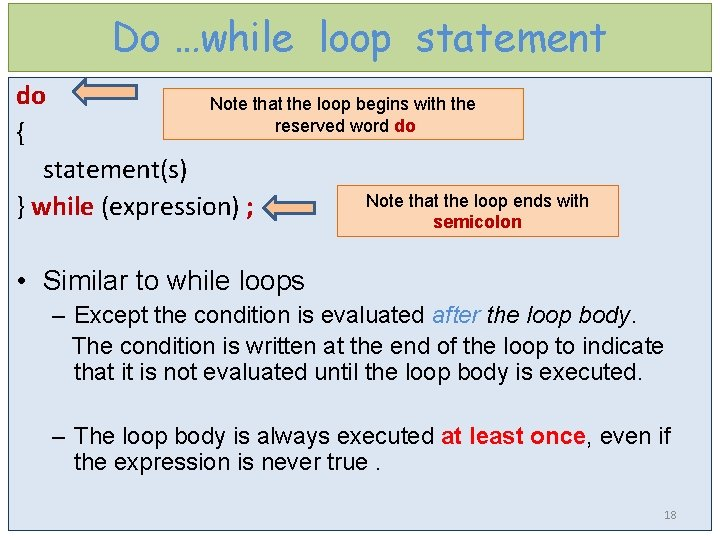Do …while loop statement do Note that the loop begins with the reserved word do { statement(s) Note that the loop ends with } while (expression) ; semicolon • Similar to while loops – Except the condition is evaluated after the loop body. The condition is written at the end of the loop to indicate that it is not evaluated until the loop body is executed. – The loop body is always executed at least once, even if the expression is never true. 18Do …while loop execution 19Do …while loop example 1 Waiting for legal input // program statement before the do…while loop System. out. print( "Please, enter a positive number: “ ); do { int num = reader. next. Int(); if (num <= 0) System. out. println( “Input error ! Try again “ ); } while ( num <= 0 ); // program statements after the do…while loop 20Do …while loop example 2 This program section reads an integer and reverses its digit mathematically. int revers. Num = 0; // reversed number System. out. println("enter an integer : "); int num = reader. next. Int( ); // input variable do { int last. Digit = num % 10; revers. Num = (revers. Num*10 )+ last. Digit; num = num / 10; } while (num > 0); System. out. println(" That number reversed is “ + revers. Num); 21Do …while loop example 3 This program section reads an integer and …? int x = 1; // ? System. out. println("enter an integer : "); int num = reader. next. Int( ); // input variable do { x *= num; } while ( -- num >= 1); System. out. println(" x= “ + x ); 22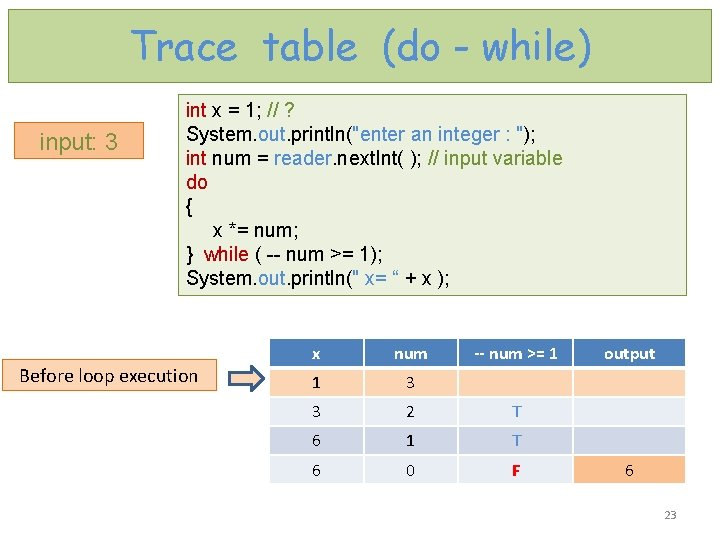Trace table (do - while) input: 3 int x = 1; // ? System. out. println("enter an integer : "); int num = reader. next. Int( ); // input variable do { x *= num; } while ( -- num >= 1); System. out. println(" x= “ + x ); Before loop execution x num -- num >= 1 1 3 3 2 T 6 1 T 6 0 F output 6 23Fibonacci series • The Fibonacci sequence is named after Leonardo of Pisa, who was known as Fibonacci. • In mathematics, the Fibonacci numbers or Fibonacci series or Fibonacci sequence are the numbers in the following integer sequence: n: 0 1 2 3 4 5 6. . . Number of element in the series : 0 1 1 2 3 5 8. . . 0 n= 0 = 1 n=1 + n >1 24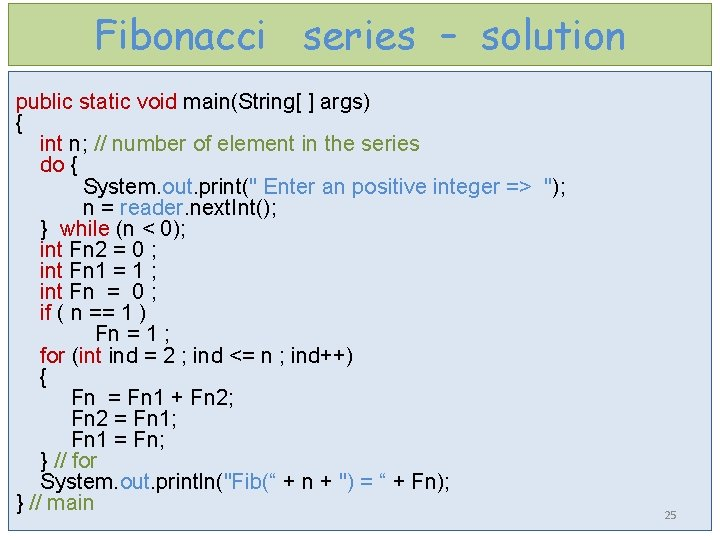Fibonacci series – solution public static void main(String[ ] args) { int n; // number of element in the series do { System. out. print(" Enter an positive integer => "); n = reader. next. Int(); } while (n < 0); int Fn 2 = 0 ; int Fn 1 = 1 ; int Fn = 0 ; if ( n == 1 ) Fn = 1 ; for (int ind = 2 ; ind <= n ; ind++) { Fn = Fn 1 + Fn 2; Fn 2 = Fn 1; Fn 1 = Fn; } // for System. out. println("Fib(“ + n + ") = “ + Fn); } // main 25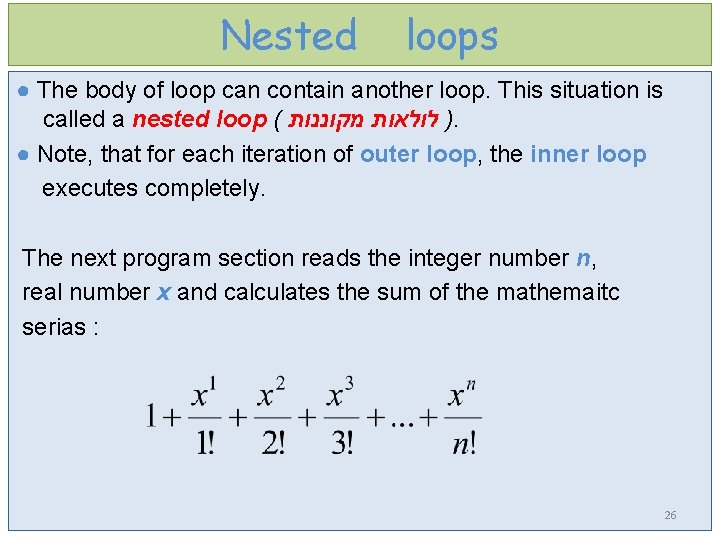Nested loops ● The body of loop can contain another loop. This situation is called a nested loop ( ) לולאות מקוננות. ● Note, that for each iteration of outer loop, the inner loop executes completely. The next program section reads the integer number n, real number x and calculates the sum of the mathemaitc serias : 26Nested loops example 1 double sum = 1. 0; // sum of series int f; // n factorial double h; // x degree int i = 1; // loop counter System. out. print( "enter an integer : “ ); int n = reader. next. Int(); System. out. print( "enter real number : “ ); double x = reader. next. Double(); while (i <= n) { outer loop ( חיצונית לולאה ) f = 1; h = 1. 0; for (int j = 1; j <= i ; j++) { f*= j; // calculates factorial inner loop ( פנימית לולאה ) h*= x; // calculates x degree } // for sum+=h/f; i++; } // while System. out. print( "sum = : "+sum ); 27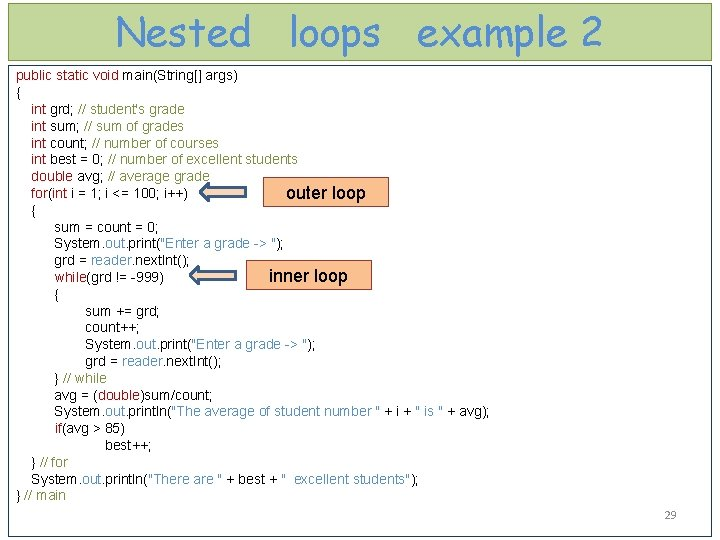Nested loops example 2 public static void main(String[] args) { int grd; // student's grade int sum; // sum of grades int count; // number of courses int best = 0; // number of excellent students double avg; // average grade for(int i = 1; i <= 100; i++) outer loop { sum = count = 0; System. out. print("Enter a grade -> "); grd = reader. next. Int(); inner loop while(grd != -999) { sum += grd; count++; System. out. print("Enter a grade -> "); grd = reader. next. Int(); } // while avg = (double)sum/count; System. out. println("The average of student number " + i + " is " + avg); if(avg > 85) best++; } // for System. out. println("There are " + best + " excellent students"); } // main 29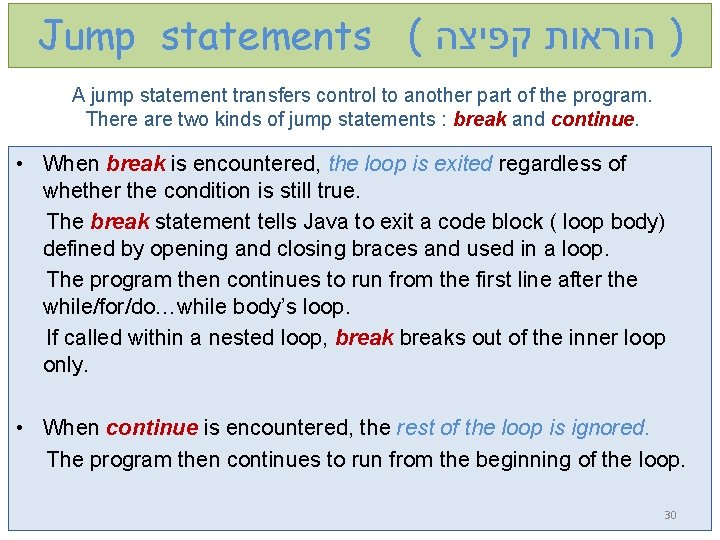Jump statements ( ) הוראות קפיצה A jump statement transfers control to another part of the program. There are two kinds of jump statements : break and continue. • When break is encountered, the loop is exited regardless of whether the condition is still true. The break statement tells Java to exit a code block ( loop body) defined by opening and closing braces and used in a loop. The program then continues to run from the first line after the while/for/do…while body’s loop. If called within a nested loop, breaks out of the inner loop only. • When continue is encountered, the rest of the loop is ignored. The program then continues to run from the beginning of the loop. 30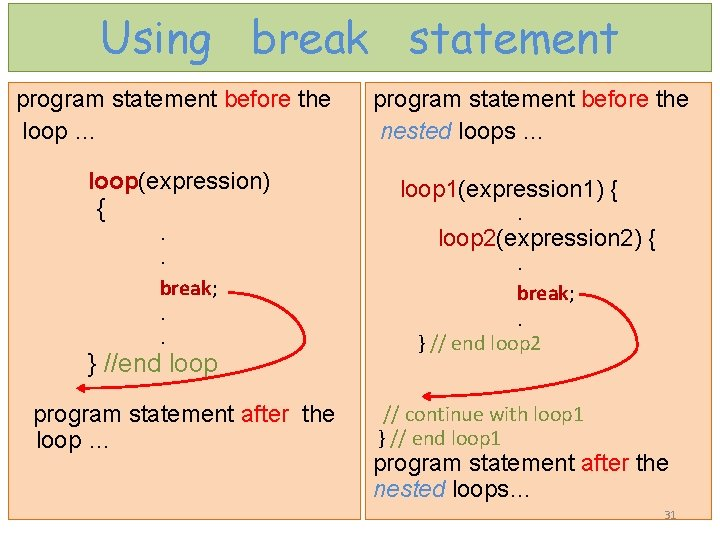Using break statement program statement before the loop … program statement before the nested loops … loop(expression) { loop 1(expression 1) { . . break; . . } //end loop program statement after the loop … . loop 2(expression 2) { . break; . } // end loop 2 // continue with loop 1 } // end loop 1 program statement after the nested loops… 31Using continue statement program statement before the loop… loop ( expression ) { . . continue; . . } // loop Ignore executing rest of the loop’s body in this iteration program statements after the loop… // continue with the program 32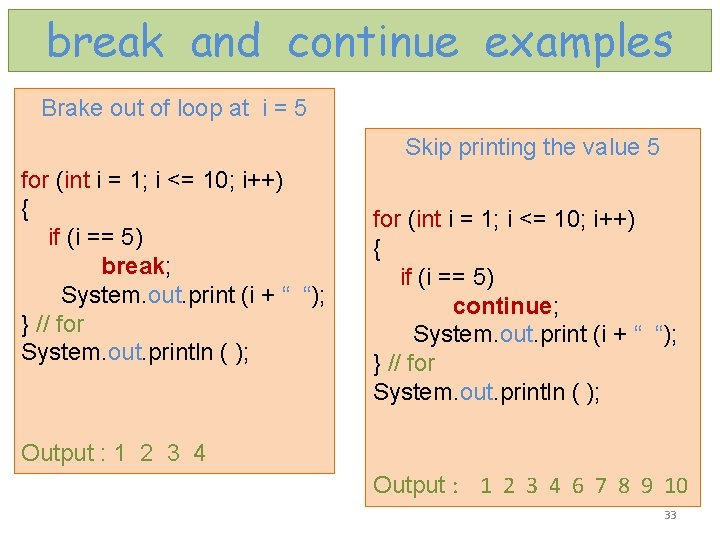break and continue examples Brake out of loop at i = 5 Skip printing the value 5 for (int i = 1; i <= 10; i++) { if (i == 5) break; System. out. print (i + “ “); } // for System. out. println ( ); for (int i = 1; i <= 10; i++) { if (i == 5) continue; System. out. print (i + “ “); } // for System. out. println ( ); Output : 1 2 3 4 6 7 8 9 10 33Caution ! ● Similarly, the following while loop is wrong: int i = 0; Error ! while (i < 10); { System. out. println("i is " + i); i++; } ● In the case of the do…while loop, the following semicolon is needed to end the loop. int i = 0; do { System. out. println("i is " + i); i++; } while (i < 10); Correct ! 34